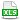HomeTechniques and Tips@RISK DistributionsSelecting Exactly Two Items (Two Numbers Guaranteed Different)

# 3.43. Selecting Exactly Two Items (Two Numbers Guaranteed Different)

Applies to:
@RISK, all versions

Question:
I have N items, and every iteration I need to select exactly two of them. Let's say N = 25, for example. I can't just use two RiskIntUniform(1,25) because they might come up with the same number in a given iteration. I need two unique items in every iteration. (I'm not worried about repetitions between iterations, just that the two numbers I get in any particular iteration are always different from each other.)

Response:
The attached workbook shows two methods to accomplish this. Each method varies the two selections independently but guarantees that they'll never be equal in any one iteration. You can tap F9 repeatedly to see how each method selects two numbers, and each time the two are different. If you run a simulation, it will count the number of occurrences where the two numbers are the same; that is zero because they are always different, as desired.

In Method A, you use RiskIntUniform(1,25) to select the first one. Therefore, 24 items have not been selected as the first item, so you use RiskIntUniform(1,24) to help you find the second one. Specifically, to find the second one you add the two RiskIntUniform functions together and then, if the total is greater than N, you subtract N.  For example, suppose that on one iteration you get 16 from RiskIntUniform(1,25) and 19 from RiskIntUniform(1,24). Then your second selection is number 35 ( = 16+5–10).

Method A is fairly straightforward, but it has a small problem: the 25 numbers are not quite equally likely to occur over the course of the whole simulation. (See the 'Method A Results worksheet in the attached workbook.) Why does this happen? Adding two independent distributions, as Method A does, tends to lose some of the advantage you normally get from the stratified sampling method of Latin Hypercube.

Method B overcomes this problem, but at the cost of some complexity. Start with the number of ways to draw two numbers from N without replacement: that is N(N–1)/2.  For N = 25, there are 300 possibilities, which you can think of as numbered from 1 to 300. Therefore, Method B uses a RiskIntUniform(1,300). In each iteration, the integer value is "decoded" to a pair of unique integers 1–25. (If you look at the formulas, you'll see some pretty involved algebra.)

Now the bumpiness of the results of Method A is gone. On the 'Method B Results' sheet, you can see that all 25 numbers come up exactly the same number of times.

Looking at the formulas on the 'TWO METHODS' sheet, you might be suspicious of the formulas for first and second selection with Method B. Maybe they work for 25 items but not for other numbers of items? That's the purpose of the last sheet, 'Method B Verify'. It shows that, for any number of items from 3 to 100, the RiskIntUniform of Method B does cover all possible draws of two different numbers.

last edited: 2014-05-30

•SelectExactlyTwo.xls